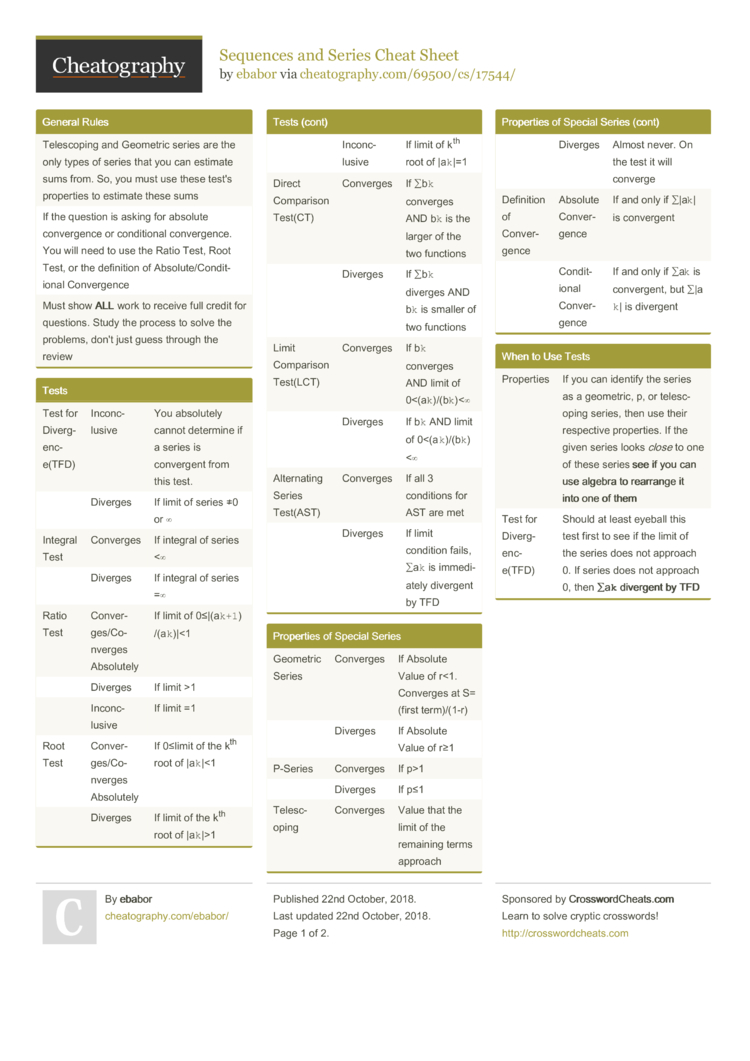# Sequences and Series Cheat Sheet by ebabor

Calculus 2 Review Sheet

### General Rules

 Telesc­oping and Geometric series are the only types of series that you can estimate sums from. So, you must use these test's properties to estimate these sums If the question is asking for absolute conver­gence or condit­ional conver­gence. You will need to use the Ratio Test, Root Test, or the definition of Absolu­te/­Con­dit­ional Conver­gence Must show ALL work to receive full credit for questions. Study the process to solve the problems, don't just guess through the review

### Tests

 Test for Diverg­enc­e(TFD) Inconc­lusive You absolutely cannot determine if a series is convergent from this test. Diverges If limit of series ≠0 or ∞ Integral Test Converges If integral of series <∞ Diverges If integral of series =∞ Ratio Test Conver­ges­/Co­nverges Absolutely If limit of 0≤|(a`­k+1`) /(a`k`­)|<1 Diverges If limit >1 Inconc­lusive If limit =1 Root Test Conver­ges­/Co­nverges Absolutely If 0≤limit of the kth root of |a`k`|­<1 Diverges If limit of the kth root of |a`k`|­>1 Inconc­lusive If limit of kth root of |a`k`|=1 Direct Comparison Test(CT) Converges If ∑b`k` converges AND b`k` is the larger of the two functions Diverges If ∑b`k` diverges AND b`k` is smaller of two functions Limit Comparison Test(LCT) Converges If b`k` converges AND limit of 0<(­a`k­`)/­(b`­k`)­<∞ Diverges If b`k` AND limit of 0<(­a`k­`)/­(b`­k`)­<∞ Altern­ating Series Test(AST) Converges If all 3 conditions for AST are met Diverges If limit condition fails, ∑a`k` is immedi­ately divergent by TFD

### Properties of Special Series

 Geometric Series Converges If Absolute Value of r<1. Converges at S=(first term)/­(1-r) Diverges If Absolute Value of r≥1 P-Series Converges If p>1 Diverges If p≤1 Telesc­oping Converges Value that the limit of the remaining terms approach Diverges Almost never. On the test it will converge Definition of Conver­gence Absolute Conver­gence If and only if ∑|a`k`| is convergent Condit­ional Conver­gence If and only if ∑a`k` is conver­gent, but ∑|a`k`| is divergent

### When to Use Tests

 Properties If you can identify the series as a geometric, p, or telesc­oping series, then use their respective proper­ties. If the given series looks close to one of these series see if you can use algebra to rearrange it into one of them Test for Diverg­enc­e(TFD) Should at least eyeball this test first to see if the limit of the series does not approach 0. If series does not approach 0, then ∑a`k` divergent by TFD Comparison Tests(CT and LCT) ONLY POSITIVE TERMS! If you can tell if the series has negative terms,­((-­1)k or sin/cos), do not use this test. If series has is rational and has a root in the denomi­nator, compare with a p-series. |a`k`| gives use absolute conver­gence Altern­ating Series Test(AST) Series with (-1)k can be testes with AST Integral Test ONLY POSITIVE TERMS! If you can look at the function and easily take the integral, it is probably good to use this test Ratio Test If series contains: k!, or powers and expone­ntials, almost guaranteed to use ratio test Root Test If the entire series can be written to the kth power, you can use the root test

### Integral Test

 Condit­ions: 1. f(x) is positive on its interval 2. f(x) is continuous on its interval 3. f(x) decreasing as x->∞ (deriv­ative is negative) * Must change a`k` to a function in order to take derivative * Integral starts off from k to ∞, so you must change the integral to k to t with limit as t->∞ * Answer you get is not where the ∑a`k` converges

### Altern­ating Series Test

 Condit­ions: 1. b`k`>0 2. b`k`≥b­`k+1` 3. limit of b`k`=0 * If ∑b`k` fits all three condit­ions, ∑a`k` convergent by AST * If 3rd condition fails, ∑a`k` is divergent by TFD * If series contains (-m)k, pull (-1)k out and keep mk in b`k`

### Integral Remainder

 Known nth Value Solving for S`n`(sum of the series approx­ima­tion) Plug n into the series Solving for R`n` (appro­xim­ation of the remainder) Solve integral from n to ∞ Known Error Bound Set the integral of f(x) from n to ∞ less than error bound. Once solved, answer will be given in terms of n<#. Must round up the number since series only use integers and if you rounded down, the value of the integral would be larger than the error bound

### Altern­ating Series Remainder

 Known Error Bound Set error bound less than b`n+1`. Solve for a #>n, round up n to next highest whole number If the inequality is very difficult to solve, the use of a table, shown below, is accept­able. When the middle column, b`n+1`, is less than third column, error bound, then that value is you final answer for n. Since the original variable in the equation is k, and k=n+1, then the value of the final term you can stop on to reach your error bound will be k nth term b`n+1` Error Bound2 Pages
//media.cheatography.com/storage/thumb/ebabor_sequences-and-series.750.jpg

PDF (recommended)